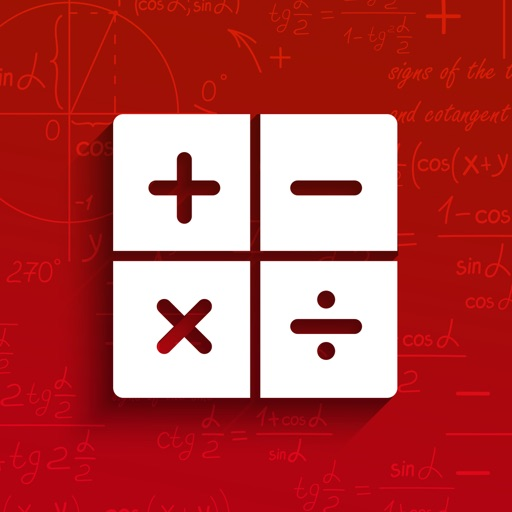## Algebra Math Solver App is your best companion for solving your mathematical problems and equations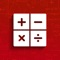# Algebra Math Solver

by Position Mobile Ltd SEZC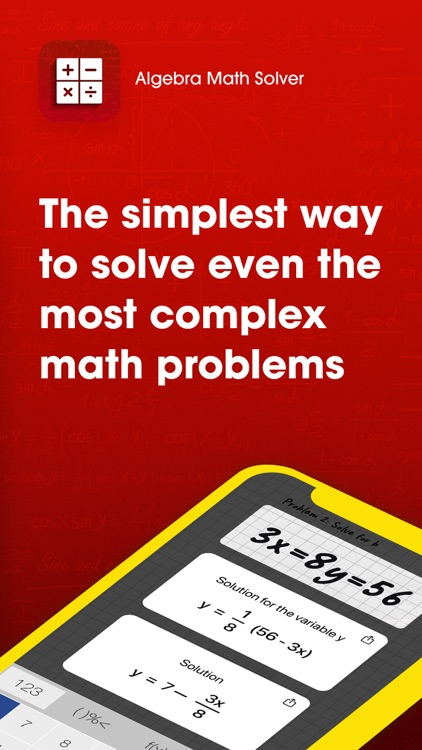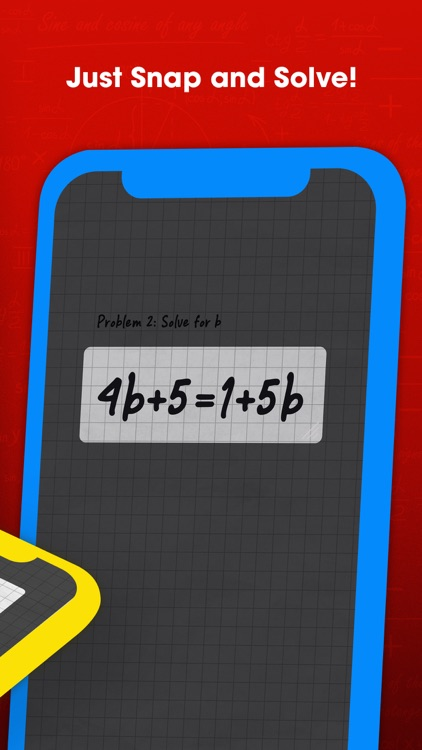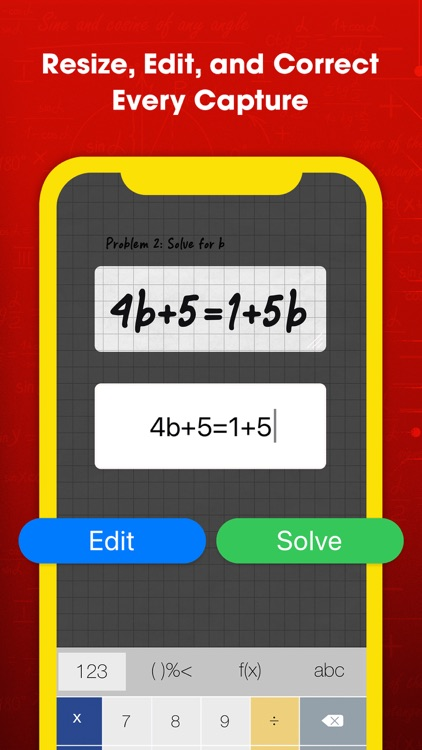Algebra Math Solver App is your best companion for solving your mathematical problems and equations. From easier algebra to the most complex calculus function equations, Algebra Math Solver provides you accurate solutions that you can trust. You can even take photos of your complex math equations and problems and the app will solve them for you! Once we solve the problem, the app will teach you how it was solved so you can understand the equation so you can repeat the mathematics steps yourself for future math solutions. Achieve perfect math homework and obtain higher grades on your mathematics tests and quizzes.### App Details

Version
24.7
Rating
(6144)
Size
68Mb
Genre
Education
Last updated
July 3, 2022
Release date
September 4, 2020

### App Screenshots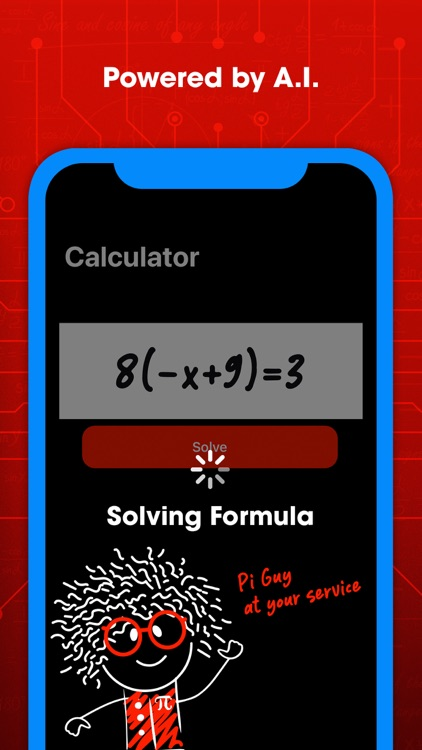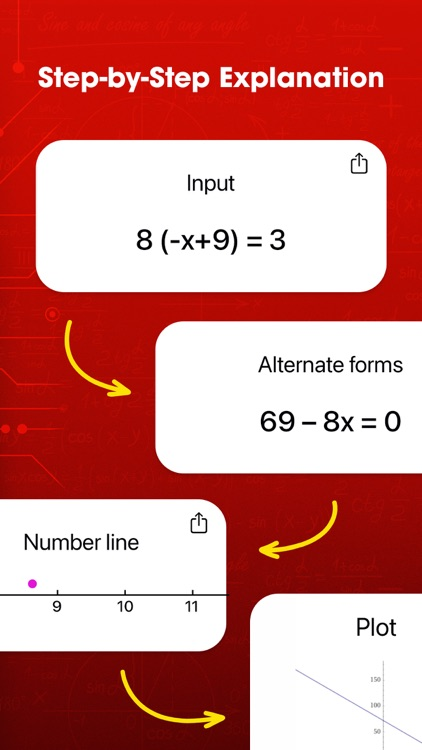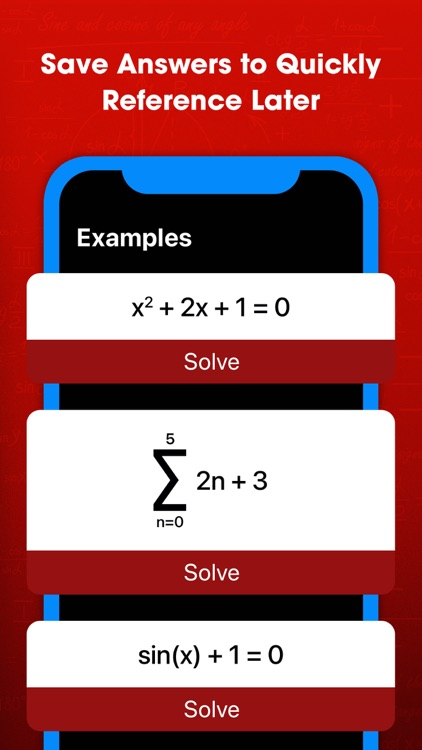### App Store Description

Algebra Math Solver App is your best companion for solving your mathematical problems and equations. From easier algebra to the most complex calculus function equations, Algebra Math Solver provides you accurate solutions that you can trust. You can even take photos of your complex math equations and problems and the app will solve them for you! Once we solve the problem, the app will teach you how it was solved so you can understand the equation so you can repeat the mathematics steps yourself for future math solutions. Achieve perfect math homework and obtain higher grades on your mathematics tests and quizzes.

Math is important in everyday life and used everywhere and now the process is easier than ever:

- Take a picture of your handwritten math problem or enter it manually using our mathematical keyboard. Instantly, the mathematics engine solves the problem for you.

- View the results and the mathematic steps to solve the problem. For a better understanding, we provide visual representations as well for certain math problems such as equations and calculus.

- You can even save your results for future use and viewing!

Math can be fun and we are making it easier for you! Get the Algebra Math Solver App now!

------------------------------------------

Algebra Math Solver App Premium Subscription

------------------------------------------

Join "Algebra Math Solver App Premium Features Club" to get:

- Unlimited math solutions and step by step details on your math exercises, equations and problems

Algebra Math Solver App has different subscription plans.

Subscription automatically renews unless auto-renew is turned off at least 24 hours before the end of the current subscription period or trial. Subscriptions starting with a trial and automatically renew for the indicated price after the trial period ends. Any unused portion of a free trial period, if offered, will be forfeited when you purchase a premium subscription during the trial period.

Subscriptions may be managed by you and auto-renewal may be turned off by going to your Account Settings after purchase ("Settings" -> "iTunes & App Store" -> "Apple ID" and select "Subscriptions")